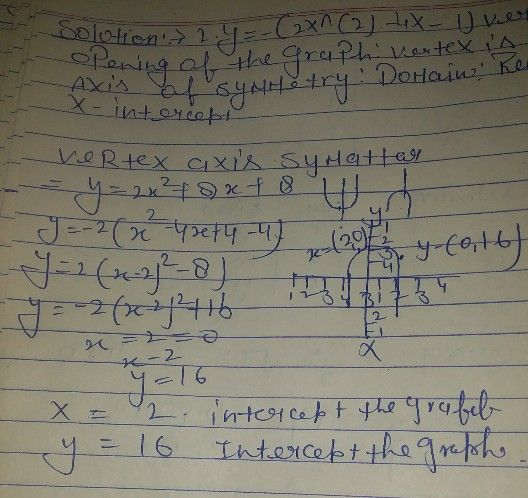Symbol
Problem$2.y=-\left(2x^{2}-4x-1\right)$ $Uertex$ Opening of the graph: Vertex is a point. Axis of $symmet\pi y$ $Domains$ Range : $x-$ $ntercept/s:$ $y-intercept/8$
1st-6th grade
Other
Search count: 115
SolutionQanda teacher - Devkiran